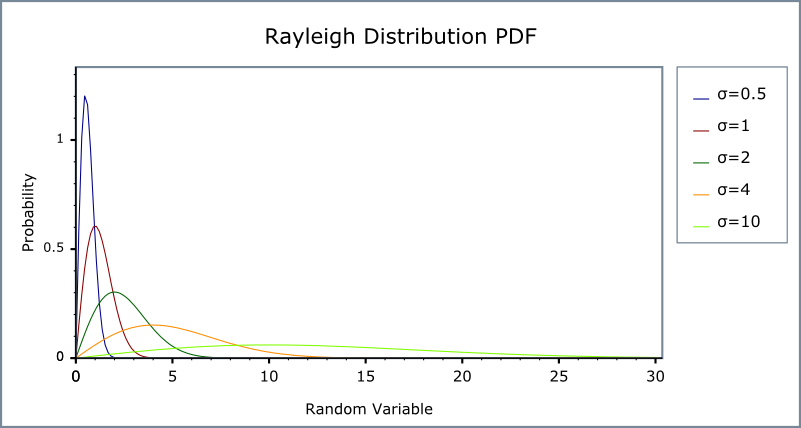#Boost C++ Libraries

...one of the most highly regarded and expertly designed C++ library projects in the world.

This is the documentation for an old version of boost. Click here for the latest Boost documentation.
##### Rayleigh Distribution

`#include <boost/math/distributions/rayleigh.hpp>`

```namespace boost{ namespace math{

template <class RealType = double,
class Policy   = policies::policy<> >
class rayleigh_distribution;

typedef rayleigh_distribution<> rayleigh;

template <class RealType, class Policy>
class rayleigh_distribution
{
public:
typedef RealType value_type;
typedef Policy   policy_type;
// Construct:
rayleigh_distribution(RealType sigma = 1)
// Accessors:
RealType sigma()const;
};

}} // namespaces
```

The Rayleigh distribution is a continuous distribution with the probability density function:

f(x; sigma) = x * exp(-x2/2 σ2) / σ2

For sigma parameter σ > 0, and x > 0.

The Rayleigh distribution is often used where two orthogonal components have an absolute value, for example, wind velocity and direction may be combined to yield a wind speed, or real and imaginary components may have absolute values that are Rayleigh distributed.

The following graph illustrates how the Probability density Function(pdf) varies with the shape parameter σ:and the Cumulative Distribution Function (cdf)##### Related distributions

The absolute value of two independent normal distributions X and Y, √ (X2 + Y2) is a Rayleigh distribution.

The Chi, Rice and Weibull distributions are generalizations of the Rayleigh distribution.

##### Member Functions
```rayleigh_distribution(RealType sigma = 1);
```

Constructs a Rayleigh distribution with σ sigma.

Requires that the σ parameter is greater than zero, otherwise calls domain_error.

```RealType sigma()const;
```

Returns the sigma parameter of this distribution.

##### Non-member Accessors

All the usual non-member accessor functions that are generic to all distributions are supported: Cumulative Distribution Function, Probability Density Function, Quantile, Hazard Function, Cumulative Hazard Function, mean, median, mode, variance, standard deviation, skewness, kurtosis, kurtosis_excess, range and support.

The domain of the random variable is [0, max_value].

##### Accuracy

The Rayleigh distribution is implemented in terms of the standard library `sqrt` and `exp` and as such should have very low error rates. Some constants such as skewness and kurtosis were calculated using NTL RR type with 150-bit accuracy, about 50 decimal digits.

##### Implementation

In the following table σ is the sigma parameter of the distribution, x is the random variate, p is the probability and q = 1-p.

Function

Implementation Notes

pdf

Using the relation: pdf = x * exp(-x2)/2 σ2

cdf

Using the relation: p = 1 - exp(-x2/2) σ2 = -expm1(-x2/2) σ2

cdf complement

Using the relation: q = exp(-x2/ 2) * σ2

quantile

Using the relation: x = sqrt(-2 * σ 2) * log(1 - p)) = sqrt(-2 * σ 2) * log1p(-p))

quantile from the complement

Using the relation: x = sqrt(-2 * σ 2) * log(q))

mean

σ * sqrt(π/2)

variance

σ2 * (4 - π/2)

mode

σ

skewness

kurtosis

kurtosis excess